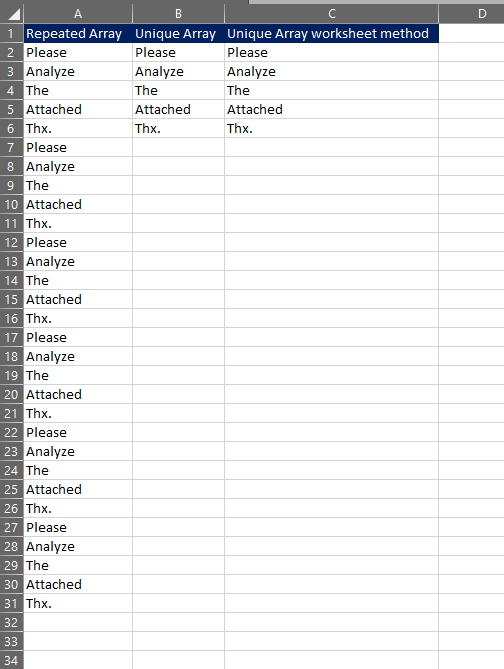top of page

# How to create a unique array and "vba filter unique values"?Now just like unicorns are unique, we sometimes want to loop through our data and filter out the unique items. I have mostly used this in combination when creating a bunch of emails where I want to attach multiple files but only wish my "To: " - email recipient to receive one email containing all the attachments. As it is slightly annoying that VBA does not have a unique array function built in I figured I would share my process using the Redim Preserve syntax for a much shorter looping script.

I have also added the code for using the worksheet unique function after the VBA example, should you have the option to run the worksheet method.

### My Worksheet### Code for my array

```Sub Unique_Array()

'N will represent the size of my array
Dim N As Integer
N = 1

Dim MyArray() As String
ReDim MyArray(N)

For Each Cell In Range("A2:A500")
If Cell <> "" Then

'looping through my array to add the unique values
For j = 1 To N

'If statement for adding the first value in my array.
If MyArray(j) = "" And j = N Then
MyArray(N) = Cell

'If statement to check whether the value exists in my array already
ElseIf MyArray(j) = Cell Then
'Exits the current array loop to go to the next value in Range A1:A500
Exit For

'If statement for adding the new unique value
ElseIf MyArray(j) <> Cell And j = N Then
'adding one for the lenght of my array.
N = N + 1

'Important to use the 'Preserve' syntax, as you will otherwise define a completely new empty array.
ReDim Preserve MyArray(N)
'Adding the value to place N, that has just been extended.
MyArray(N) = Cell

End If
Next j
End If
Next Cell

'Printing out my new unique array.
'Not really necessary to loop it out, if you have more data.
For i = 1 To N
Cells(1 + i, 2) = MyArray(i)
Next i

MsgBox ("Done")

End Sub```

### Code for using the "Unique" syntax on the worksheet.

```Sub Unique_worksheet()

'Reading in all data from cell A2 and below
Dim Rng As Range
Set Rng = Range("A2", Range("A2").End(xlDown))

'Note that we need to use the .Formula2 syntax after the cell reference.
'Excel 2019 introduced the implicit intersection operator "@", which forces formulas to print out the value into one cell.
'Since we in this case need the so called "Spill" on the other cells below we therefore need to use the .Formula2 syntax.
Range("C2").Formula2 = "=Unique(" & Rng.Address & ")" 'or .Formula2R1C1
​
MyUniqueArray = Range("C2", Range("C2").End(xlDown))

'Prints out "Thx." which our last unique value.
Debug.Print MyUniqueArray(5, 1)

End Sub```